# Create multivariate functions¶

We can create multivariate functions by different methods. One of the methods is to gather multivariate functions. The other one is to create a function having multivariate input using the tensor product of univariate functions. In this example, we present both methods.

## Description¶

We want to build some multivariate functions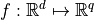.

We can do that:

• Case 1: using a finite collection of multivariate functions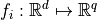,

• Case 2: using the tensor product of univariate functions,

• Case 3: using the tensor product of orthogonal univariate polynomials,

• Case 4: using the tensor product of orthogonal univariate functions.

## Case 1: Finite collection of multivariate functions¶

In that case, we have a finite collection of multivariate functions. We use the Basis class.

For example, we consider: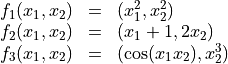import openturns as ot

f1 = ot.SymbolicFunction(['x1', 'x2'], ['x1^2', 'x2^2'])
f2 = ot.SymbolicFunction(['x1', 'x2'], ['x1+1.0', '2.0*x2'])
f3 = ot.SymbolicFunction(['x1', 'x2'], ['cos(x1*x2)', 'x2^3'])

myBasis = ot.Basis([f1, f2, f3])
f = myBasis.build(1)


## Case 2: Tensor product of univariate functions¶

In that case, the univariate functions are not necessarily orthogonal with respect to a measure.

We have the family of functions: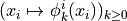for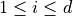.. The enumerate() function of the LinearEnumerateFunction class stores the way the multivariate basis is enumerated: enumerate(n) is a multi-index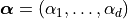such that the function number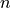is: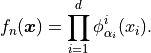We use the TensorizedUniVariateFunctionFactory class.

For example, we consider: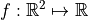, and:

• dimension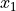: the family of monomials: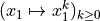,

• dimension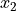: the family of Haar wavelets: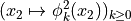.

univFuncFamily_Mon = ot.MonomialFunctionFactory()
univFuncFamily_UnivPol = ot.OrthogonalUniVariatePolynomialFunctionFactory(ot.JacobiFactory())
univFuncFamily_Haar = ot.HaarWaveletFactory()
univFuncFamily_Fourier = ot.FourierSeriesFactory()

familyColl = [univFuncFamily_Mon, univFuncFamily_UnivPol, univFuncFamily_Haar, univFuncFamily_Fourier]
enumerateFunction = ot.LinearEnumerateFunction(len(familyColl))

familyFunction = ot.TensorizedUniVariateFunctionFactory(familyColl, enumerateFunction)
k = 3
f = familyFunction.build(k)
print('input dimension = ', f.getInputDimension())
print('output dimension = ', f.getOutputDimension())

input dimension =  4
output dimension =  1


If we want to use an orthogonal univariate polynomials family, then we have to cast the family in the OrthogonalUniVariatePolynomialFunctionFactory class.

For example, we use the Jacobi orthogonal univariate polynomials family.

univFuncFamily_Jacobi = ot.OrthogonalUniVariatePolynomialFunctionFactory(ot.JacobiFactory())


## Case 3: Tensor product of orthogonal univariate polynomials¶

In that case, the univariate polynomials are orthogonal with respect to a measure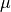.

We have the family of polynomials:for. The enumerate() function stores of the LinearEnumerateFunction class the way the multivariate basis is enumerated: enumerate(n) is a multi-index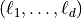such that the function numberis: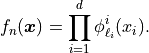We use the OrthogonalProductPolynomialFactory class.

For example, we consider :, and:

• dimension of: the family of Jacobi polynomials: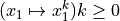,

• dimension of: the family of Hermite polynomials:.

univFuncFamily_Jacobi = ot.JacobiFactory()
univFuncFamily_Hermite = ot.HermiteFactory()
familyColl = [univFuncFamily_Jacobi, univFuncFamily_Hermite]
enumerateFunction = ot.LinearEnumerateFunction(len(familyColl))
familyFunction = ot.OrthogonalProductPolynomialFactory(familyColl, enumerateFunction)
f = familyFunction.build(3)
print('input dimension = ', f.getInputDimension())
print('output dimension = ', f.getOutputDimension())

input dimension =  2
output dimension =  1


We get the measure:

measure_Jacobi = ot.JacobiFactory().getMeasure()
measure_Hermite = ot.HermiteFactory().getMeasure()
print('Measure orthogonal to Jacobi polynomials = ', measure_Jacobi)
print('Measure orthogonal to Hermite polynomials = ', measure_Hermite)

Measure orthogonal to Jacobi polynomials =  Beta(alpha = 2, beta = 2, a = -1, b = 1)
Measure orthogonal to Hermite polynomials =  Normal(mu = 0, sigma = 1)


## Case 4: Tensor product of orthogonal univariate functions¶

In that case, the univariate functions are orthogonal with respect to a measure.

We have the family of functions:for. The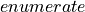function of the LinearEnumerateFunction class stores the way the multivariate basis is enumerated: enumerate(n) is a multi-indexsuch that the function number n is: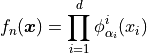We use the OrthogonalProductFunctionFactory class.

For example, we consider :, and:

• dimension: the family of Haar wavelets: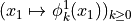,

• dimension: the family of Fourier series functions: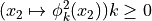.

univFuncFamily_Haar = ot.HaarWaveletFactory()
univFuncFamily_Fourier = ot.FourierSeriesFactory()
familyColl = [univFuncFamily_Haar, univFuncFamily_Fourier]
enumerateFunction = ot.LinearEnumerateFunction(len(familyColl))

familyFunction = ot.OrthogonalProductFunctionFactory(familyColl, enumerateFunction)
k = 3
f = familyFunction.build(k)


We get the measure:

measure_Haar = ot.HaarWaveletFactory().getMeasure()
measure_Fourier = ot.FourierSeriesFactory().getMeasure()
print('Measure orthogonal to Haar wavelets = ', measure_Haar)
print('Measure orthogonal to Fourier series = ', measure_Fourier)

Measure orthogonal to Haar wavelets =  Uniform(a = 0, b = 1)
Measure orthogonal to Fourier series =  Uniform(a = -3.14159, b = 3.14159)